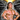# Somethinghitme

## Code, demos and ideas.

### Game Related Snippets I always Forget

April 26, 2010

When I sit down to prototype something new I always find myself looking up the same snippets to do the same types of things. I figured I might as well record them somewhere so I know an easy place to look when I do forget. All the samples will be in Javascript and should be able to be easily converted to any other language.

Distance Formula

Obviously a pretty important one, used to tell the distance between 2 objects. Can be used for simple radius collisions as well.

`` var distance = Math.sqrt((x2 - x1) *(x2-x1) + (y2 - y1) * (y2-y1));``

Move toward an object (Without rotation) with easing

`` x += (targetX-x)/speed; y += (targetY-y)/speed;``

Angle Based Movement

I always see myself needing this one, whether its for an Asteroids clone, or a follow the leader type game.

`` velX = Math.cos((angle * Math.PI / 180) * thrust); velY = Math.sin((angle * Math.PI / 180) * thrust);``

Friction

How do I make something slow down if no force is applied?

`` velX *= .98; //Any val under 1 is suitable for your needs velY *= .98``

2D Matrix

This is a pretty simple one I know.. but I always seem to forget it. This is how to store a 2d matrix in an array. And how to find a value you need.

`````` // 2d matrix\[y * width + x\] var arr = \[\], width = 10;

for(x=0; x<width; x++){ for(y=0; y<width; y++){ arr\[y * 10 + x\] = x + y; //Or whatever value you need to assign } }

//Retrieve a value from x:5, y:2 var val = arr\[2 * 10 + 5\];

//get the x and y from a 1d array var index = 12, x = index % width, // 2 y = Math.floor(index / width); // 1``````

Move towards and object at a constant speed

`````` var tx = targetX - x, ty = targetY - y, dist = Math.sqrt(tx * tx+ty * ty), rad = Math.atan2(ty,tx), angle = rad / Math.PI * 180;;

velX = (tx / dist) * thrust; velY = (ty / dist) * thrust;``````

Example

Project a point in front of an object

`` x + length * Math.cos(angle * Math.PI / 180); y + length * Math.sin(angle * Math.PI / 180);``

Get the angle between objects

`````` var x = targetX - this.x, y = targetY - this.y;

return Math.atan2(x,-y);``````

Plot points around a circle

`````` var rad = 500, total = 50, centerX = 250, centerY = 250;

for(var i = 0; i < total; i++){
ctx.beginPath(); ctx.moveTo(centerX,centerX);
var x = centerX + rad * Math.cos(2 * Math.PI * i / total),
y = centerY + rad * Math.sin(2 * Math.PI * i / total);

ctx.lineTo(x, y); ctx.stroke(); ctx.closePath();
}``````Written by Jason Brown Father, Veteran, lover of everything tech.